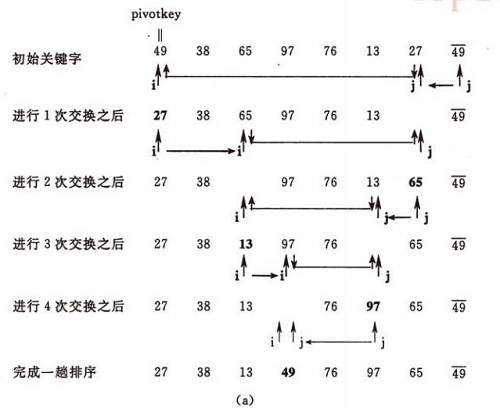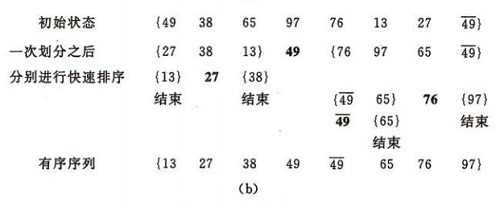# 交换排序—快速排序（Quick Sort）

java交换排序—快速排序（Quick Sort）,1）选择一个基准元素,通常选择第一个元素或者最后一个元素，通过一趟排序讲待排序的记录分割成独立的两部分，其中一部分记录的元素值均比基准元素值小。另一部分记录的 元素值比基准值大。此时基准元素在其排好序后的正确位置，然后分别对这两部分记录用同样的方法继续进行排序，直到整个序列有序。

基本思想：

1）选择一个基准元素,通常选择第一个元素或者最后一个元素,

2）通过一趟排序讲待排序的记录分割成独立的两部分，其中一部分记录的元素值均比基准元素值小。另一部分记录的 元素值比基准值大。

3）此时基准元素在其排好序后的正确位置

4）然后分别对这两部分记录用同样的方法继续进行排序，直到整个序列有序。

快速排序的示例：

（a）一趟排序的过程：（b）排序的全过程算法的实现：

递归实现：

```/*
* Java实现快速排序算法
* author:wyr
* 2016-7-14
*/
public class QuickSort {
public static void main(String[] args) {

int a[] = {3,1,5,7,2,4,9,6,10,8};
QuickSort  obj=new QuickSort();
System.out.println("初始值：");
obj.print(a);
int h=a.length-1;
obj.quickSort(a,0,h);
System.out.println("\n排序后：");
obj.print(a);
}
private  void quickSort(int[] a,int low, int high) {
if(low<high){ //如果不加这个判断递归会无法退出导致堆栈溢出异常
int middle=getMiddle(a,low,high);
quickSort(a,  0,  middle-1);          //递归对低子表递归排序
quickSort(a,   middle + 1, high);        //递归对高子表递归排序
}
}
public int getMiddle(int[] a, int low, int high){

int key = a[low];//基准元素，排序中会空出来一个位置
while(low<high){
while(low<high && a[high]>=key){//从high开始找比基准小的，与low换位置
high--;
}
a[low]=a[high];
while(low<high && a[low]<=key){//从low开始找比基准大,放到之前high空出来的位置上
low++;
}
a[high]=a[low];
}
a[low]=key;//此时low=high 是基准元素的位置，也是空出来的那个位置
return low;

}
public void print(int a[]){
for(int i=0;i<a.length;i++){
System.out.print(a[i]+" ");
}
}
}```

分析：

快速排序是通常被认为在同数量级（O(nlog2n)）的排序方法中平均性能最好的。但若初始序列按关键码有序或基本有序时，快排序反而蜕化为冒泡排序。为改进之，通常以“三者取中法”来选取基准记录，即将排序区间的两个端点与中点三个记录关键码居中的调整为支点记录。快速排序是一个不稳定的排序方法。

快速排序的改进

在本改进算法中,只对长度大于k的子序列递归调用快速排序,让原序列基本有序，然后再对整个基本有序序列用插入排序算法排序。实践证明，改进后的算法时间复杂度有所降低，且当k取值为 8 左右时,改进算法的性能最佳。算法思想如下：

```void print(int a[], int n){
for(int j= 0; j<n; j++){
cout<<a[j] <<"  ";
}
cout<<endl;
}

void swap(int *a, int *b)
{
int tmp = *a;
*a = *b;
*b = tmp;
}

int partition(int a[], int low, int high)
{
int privotKey = a[low];                 //基准元素
while(low < high){                   //从表的两端交替地向中间扫描
while(low < high  && a[high] >= privotKey) --high; //从high 所指位置向前搜索，至多到low+1 位置。将比基准元素小的交换到低端
swap(&a[low], &a[high]);
while(low < high  && a[low] <= privotKey ) ++low;
swap(&a[low], &a[high]);
}
print(a,10);
return low;
}

void qsort_improve(int r[ ],int low,int high, int k){
if( high -low > k ) { //长度大于k时递归, k为指定的数
int pivot = partition(r, low, high); // 调用的Partition算法保持不变
qsort_improve(r, low, pivot - 1,k);
qsort_improve(r, pivot + 1, high,k);
}
}
void quickSort(int r[], int n, int k){
qsort_improve(r,0,n,k);//先调用改进算法Qsort使之基本有序

//再用插入排序对基本有序序列排序
for(int i=1; i<=n;i ++){
int tmp = r[i];
int j=i-1;
while(tmp < r[j]){
r[j+1]=r[j]; j=j-1;
}
r[j+1] = tmp;
}

}

int main(){
int a = {3,1,5,7,2,4,9,6,10,8};
cout<<"初始值：";
print(a,10);
quickSort(a,9,4);
cout<<"结果：";
print(a,10);

}```2 +1

Java  源码  算法

请文明留言

0 条评论